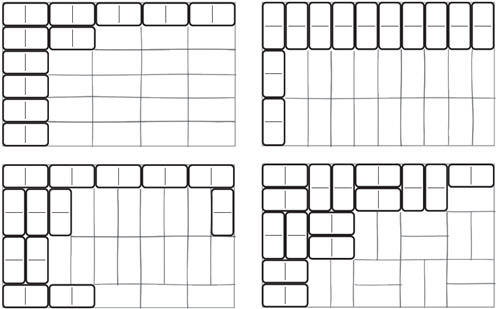Go to website

# reSolve: Multiplication: Domino Arrays

This sequence of two lessons explores multiplicative thinking through the use of arrays where all the parts of the array are not visible. The sequence encourages students to find the total number of items in an array by multiplication rather than counting by ones or skip counting. Connections between area, arrays and multiplication are also made. Each lesson is outlined in detail including curriculum links, vocabulary, materials needed, sample answers, discussion points and student resources. This sequence is part of the reSolve: Mathematics by Inquiry program.

Year level(s) Year 3
Audience Teacher
Purpose Teaching resource
Teaching strategies and pedagogical approaches Mathematics investigation
Keywords division, written calculations, mental arithmetic, multiplication

## Curriculum alignment

Curriculum connections Numeracy
Strand and focus Number, Build understanding, Apply understanding
Topics Multiplication and division
AC: Mathematics (V9.0) content descriptions
AC9M3A03
Recall and demonstrate proficiency with multiplication facts for 3, 4, 5 and 10; extend and apply facts to develop the related division facts

AC9M3N04
Multiply and divide one- and two-digit numbers, representing problems using number sentences, diagrams and arrays, and using a variety of calculation strategies

Numeracy progression Multiplicative strategies (P5)
Number patterns and algebraic thinking (P4)